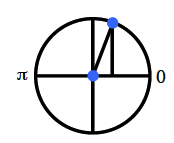### Home > PC3 > Chapter 6 > Lesson 6.1.3 > Problem6-50

6-50.

Many times when solving trigonometric equations, the solutions are not convenient values on the unit circle where the answers are exact. For example, $2\sin(x)=1$ yields $x=\sin−1(\frac{1}{2})$ and the exact solution between $-\frac{\pi}{2}$ and $\frac { \pi } { 2 }$ is $\frac { \pi } { 6 }$. The other solution between $0$ and $2π$ is $\frac { 5 \pi } { 6 }$. But what happens when trying to solve $5\sin(x)=4$?

1. Solve $5\sin(x)=4$ for $x$ and give a decimal approximation.

$\sin(x)=\frac{4}{5}$

2. Is this the only solution between $0$ and $2π$? Use what you know about the symmetry of the sine function and the unit circle to determine the other solution between $0$ and $2π$.

Use the unit circle and symmetry.

$\text{Where else is }\sin(x)=\frac{4}{5}?$

How can you write this?